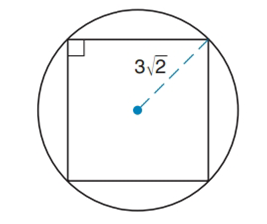Chapter 4.3, Problem 30EElementary Geometry For College St...

7th Edition
Alexander + 2 others
ISBN: 9781337614085

Solutions

Chapter
SectionElementary Geometry For College St...

7th Edition
Alexander + 2 others
ISBN: 9781337614085
Textbook Problem

Find the perimeter of the square shown.To determine

To find:

The perimeter of the square.

Explanation

Calculation:

Consider the square ABCD and the inscribing circle whose radius (OB) is 32 units.

BD is the diameter of circle.

Let ‘O’ be the center of the circle.

Step 1:

To find DB:

DB=OD+OB=r+r=32+32DB=62

In right ABD, let AB = AD = a

By using Pythagorean Theorem

Still sussing out bartleby?

Check out a sample textbook solution.

See a sample solution

The Solution to Your Study Problems

Bartleby provides explanations to thousands of textbook problems written by our experts, many with advanced degrees!

Get Started

Compute C5,2.

Understanding Basic Statistics

11. What is the mean of the scores in Problem 8?

Mathematical Applications for the Management, Life, and Social Sciences

Prove Property 3 of integrals.

Single Variable Calculus: Early Transcendentals

True or False: The vector function is continuous at t = 3.

Study Guide for Stewart's Multivariable Calculus, 8th

Area In Exercises 57 and 58, find the area of the region. x=3siny=2cos22

Calculus: Early Transcendental Functions (MindTap Course List)

∫ sin 3x cos 6x dx =

Study Guide for Stewart's Single Variable Calculus: Early Transcendentals, 8th